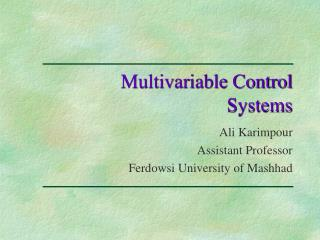# Multivariable Control Systems - PowerPoint PPT PresentationDownload PresentationMultivariable Control Systems

Multivariable Control SystemsDownload Presentation## Multivariable Control Systems

- - - - - - - - - - - - - - - - - - - - - - - - - - - E N D - - - - - - - - - - - - - - - - - - - - - - - - - - -
##### Presentation Transcript

1. Multivariable Control Systems Ali Karimpour Assistant Professor Ferdowsi University of Mashhad

2. Chapter 6 Introduction to Decoupling Control Topics to be covered include: • Decoupling • Pre and post compensators and the SVD controller • Decoupling by State Feedback • Diagonalcontroller (decentralized control)

3. Introduction We see that every input controls more than one output and that every output is controlled by more than one input. Because of this phenomenon, which is called interaction, it is generally very difficult to control a multivariable system.

4. Decoupling Decoupling Definition 6-1 A multivariable system is said to be decoupled if its transfer-function matrix is diagonal and nonsingular. A conceptually simple approach to multivariable control is given by a two-steps procedure in which 1. We first design a compensator to deal with the interactions in G(s) and 2. Then design a diagonal controller using methods similar to those for SISO systems.

5. 1. We first design a compensator to deal with the interactions in G(s) and Decoupling Decoupling • Dynamic decoupling It usually refers to an inverse-based controller. • Steady-state decoupling This may be obtained by selecting a constant pre compensator • Approximate decoupling at frequency ω0 This is usually obtained by choosing a constant pre compensator

6. Decoupling The idea of using a decoupling controller is appealing, but there are several difficulties. • We cannot in general choose Gs freely. For example, Ws(s) must not cancel any • RHP-zeros and RHP poles in G(s) • As we might expect, decoupling may be very sensitive to modeling errors and • uncertainties. c. The requirement of decoupling may not be desirable for disturbance rejection. One popular design method, which essentially yields a decoupling controller, is the internal model control (IMC) approach (Morari and Zafiriou). Another common strategy, which avoids most of the problems just mentioned, is to use partial (one-way) decoupling where Gs(s)is upper or lower triangular.

7. Pre and post compensators and the SVD controller The pre compensator approach may be extended by introducing a post compensator The overall controller is then

8. Decoupling by State Feedback In this section we consider the decoupling of a control system in state space representation. Let The transfer function matrix is We shall derive in the following the condition on G(s) under which the system can be decoupled by state feedback.

9. Theorem 6-1 A system represented by with the transfer function matrix G(s) can be decoupled by state feedback of the form if and only if the constant matrix E is nonsingular Decoupling by State Feedback Furthermore and Proof: See “Linear system theory and design” Chi-Tsong Chen

10. Decoupling by State Feedback Example 6-2 Use state feedback to decouple the following system. Solution: Transfer function of the system is The differences in degree of the first row of G(s)are 1 and 2, hence d1=1 and The differences in degree of the second row of G(s)are 2 and 1, hence d2=1 and

11. Decoupling by State Feedback Solution (continue): Now E is unitary matrix and clearly nonsingular so decoupling by state feedback is possible and The decoupled system is Exercise 1: Derive the corresponding decoupled transfer function matrix.

12. Diagonal controller (decentralized control) Another simple approach to multivariable controller design is to use a diagonal or block diagonal controller K(s). This is often referred to as decentralized control. Clearly, this works well if G(s) is close to diagonal, because then the plant to be controlled is essentially a collection of independent sub plants, and each element in K(s)may be designed independently. However, if off diagonal elements in G(s) are large, then the performance with decentralized diagonal control may be poor because no attempt is made to counteract the interactions.

13. Diagonal controller (decentralized control) The design of decentralized control systems involves two steps: 1_ The choice of pairings (control configuration selection) 2_ The design (tuning) of each controller ki(s) In this section we provide two useful rules for pairing inputs and outputs. 1_ To avoid instability caused by interactions in the crossover region one should prefer pairings for which the RGA matrix in this frequency range is close to identity. 2_ To avoid instability caused by interactions at low frequencies one should avoid pairings with negative steady state RGA elements.

14. Diagonal controller (decentralized control) Example 6-3 Select suitable pairing for the following plant Solution: RGA of the system is